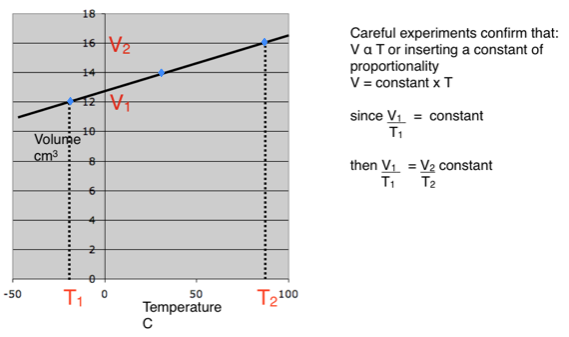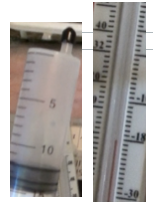### Charles’s Law

Charles’s Law

Like Boyle’s Law, when Charles’s Law was written it was empirical. It states that:

The volume a a fixed mass of gas is directly proportional to the temperature providing that the pressure remains constant.

If we take a fixed mass of gas at constant pressure and vary the temperature then the volume changes in direct proportion:When we use this equation the temperature must be in Kelvin.     The kelvin temperature is based on absolute zero.    The kelvin temperature equals the celcius temperature plus 273.

e.g.        0 oC    =  273K         27 oC  =  300K          100 oC = 373K

When a gas is heated the energy is contained in the increasing kinetic energy of the molecules. Those molecules hit the surfaces harder and therefore exert a greater force and a greater pressure. If the pressure is to be kept constant then the gas must expand so that the impacts are less frequent, therefore, at a constant pressure the volume is directly proportional to the rise in temperature.

You can download a PDF copy of these notes here:   Charles law

This idea can be extended to estimate a value of absolute zero temperature - see further video and notes below.

### Finding the value of Absolute Zero

A fixed mass of gas is trapped in a plastic syringe. The syringe is then placed in a bath of water which is heated. The volume is then measured and plotted against temperature, producing the straight line graph.

Now imagine that we then cooled the gas to very low temperatures.

As a gas is cooled the molecules move more and more slowly and the volume falls, still following a straight line. If that continues there must be, in theory at least, a point at which the molecules stop altogether.

If they stop then they have no more energy to lose and they are at the lowest possible temperature - absolute zero. At this point the volume of the gas will be insignificant.